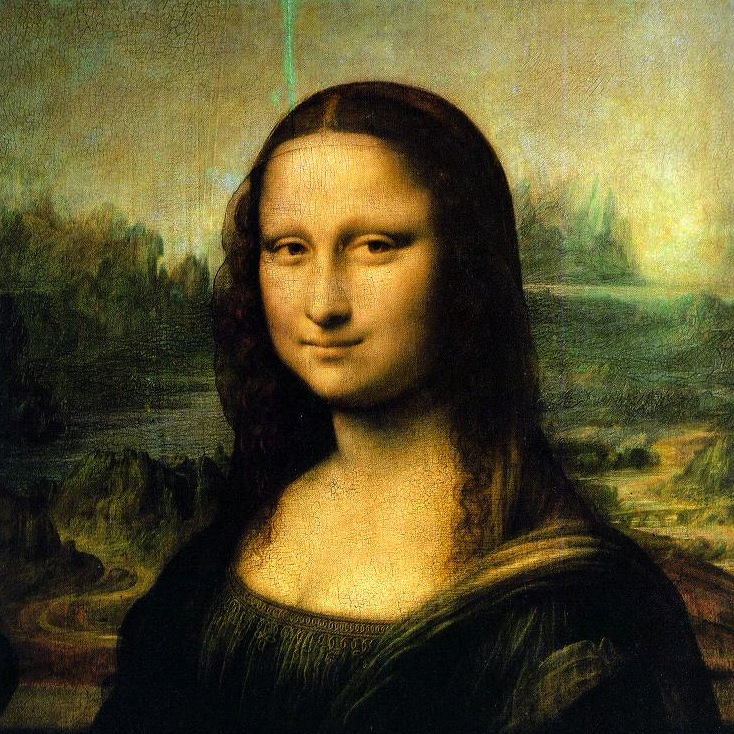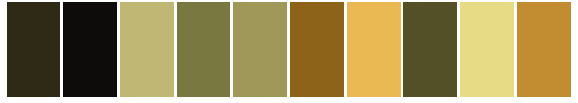## Extracting colorschemes from images

You can extract a colorscheme from an image. For example, here's an image of a famous painting:Use the extract() function to create a color scheme from the original image:

using ColorSchemeTools
monalisa = extract("monalisa.jpg", 10, 15, 0.01; shrink=2)

which in this example creates a 10-color ColorScheme object (using 15 iterations and with a tolerance of 0.01; the image can be reduced in size, here by 2, before processing, to save time).ColorSchemes.ColorScheme(ColorTypes.RGB{Float64}[
RGB{Float64}(0.0406901,0.0412985,0.0423865),
RGB{Float64}(0.823493,0.611246,0.234261),
RGB{Float64}(0.374688,0.363066,0.182004),
RGB{Float64}(0.262235,0.239368,0.110915),
RGB{Float64}(0.614806,0.428448,0.112495),
RGB{Float64}(0.139384,0.124466,0.0715472),
RGB{Float64}(0.627381,0.597513,0.340734),
RGB{Float64}(0.955276,0.775304,0.37135),
RGB{Float64}(0.497517,0.4913,0.269587),
RGB{Float64}(0.880421,0.851357,0.538013),
RGB{Float64}(0.738879,0.709218,0.441082)
], "", "")

(Extracting color schemes from images may require you to install image importing and exporting abilities. These are platform-specific. On Linux/UNIX, ImageMagick.jl can be used for importing and exporting images. Use QuartzImageIO.jl on macOS.)

ColorSchemeTools.extractFunction
extract(imfile, n=10, i=10, tolerance=0.01; shrink=n)

extract() extracts the most common colors from an image from the image file imfile by finding n dominant colors, using i iterations. You can (and probably should) shrink larger images before running this function.

Returns a ColorScheme.

## Sorting color schemes

Use sortcolorscheme() to sort a scheme non-destructively in the LUV color space:

using ColorSchemes
sortcolorscheme(ColorSchemes.leonardo)
sortcolorscheme(ColorSchemes.leonardo, rev=true)

The default is to sort colors by their LUV luminance value, but you could try specifying the :u or :v LUV fields instead (sorting colors is another problem domain not really addressed in this package...):

sortcolorscheme(ColorSchemes.leonardo, :u)
ColorSchemeTools.sortcolorschemeFunction
sortcolorscheme(colorscheme::ColorScheme, field; kwargs...)

Sort (non-destructively) a colorscheme using a field of the LUV colorspace.

The less than function is lt = (x,y) -> compare_colors(x, y, field).

The default is to sort by the luminance field :l but could be by :u or :v.

Returns a new ColorScheme.

## Weighted colorschemes

Sometimes an image is dominated by some colors with others occurring less frequently. For example, there may be much more brown than yellow in a particular image. A weighted colorscheme derived from this image can reflect this. You can extract both a set of colors and a set of numerical values or weights that indicate the relative proportions of colors in the image.

cs, wts = extract_weighted_colors("monalisa.jpg", 10, 15, 0.01; shrink=2)

The ColorScheme is now in cs, and wts holds the various weights of each color:

wts

10-element Array{Float64,1}:
0.0521126446851636
0.20025391828582884
0.08954703056671294
0.09603605342678319
0.09507086696018234
0.119987526821047
0.08042973071297582
0.08863381567908292
0.08599068966285295
0.09193772319937041

With the ColorScheme and the weights, you can make a new color scheme in which the more common colors take up more space in the scheme:

len = 50
colorscheme_weighted(cs, wts, len)

Or in one go:

colorscheme_weighted(extract_weighted_colors("monalisa.jpg" # ...

Compare the weighted and unweighted versions of schemes extracted from the Hokusai image "The Great Wave":ColorSchemeTools.extract_weighted_colorsFunction
extract_weighted_colors(imfile, n=10, i=10, tolerance=0.01; shrink = 2)

Extract colors and weights of the clusters of colors in an image file. Returns a ColorScheme and weights.

Example:

pal, wts = extract_weighted_colors(imfile, n, i, tolerance; shrink = 2)
ColorSchemeTools.colorscheme_weightedFunction
colorscheme_weighted(colorscheme, weights, length)

Returns a new ColorScheme of length length (default 50) where the proportion of each color in colorscheme is represented by the associated weight of each entry.

Examples:

colorscheme_weighted(extract_weighted_colors("hokusai.jpg")...)
colorscheme_weighted(extract_weighted_colors("filename00000001.jpg")..., 500)